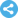International Journal of Mathematical Analysis and Applications
Manuscript Information

Exponential Attractor For a class of Higher-Order Coupled Kirchhoff-type Equations
International Journal of Mathematical Analysis and Applications
Vol.5 , No. 3, Publication Date: Jun. 1, 2018, Page: 49-57
646 Views Since June 1, 2018, 273 Downloads Since Jun. 1, 2018Paper in PDF (255K)Follow on

Authors

  Guoguang Lin, Department of Mathematics, University of Yunnan, Kunming, China.  Sanmei Yang, Department of Mathematics, University of Yunnan, Kunming, China.

Abstract

This paper mainly studies a class of higher-order coupled Kirchhoff-type equations with strongly damping and nonlinear source terms. In the process of research, first of all, Lipschitz property of the nonlinear semi-group related to the initial boundary value problem is proved by applying the Young inequality, Poincare inequality, mean value theorem, Gronwall’s inequality and so on. Then, the Discrete Squeezing property of the problem is proved by applying the boundedness of this problem in infinite dimensional space and making some changes on the left hand side of the inequality to be proved. Finally, the existence of exponential attractor is proved by using the Lipschitz property, Discrete Squeezing property and other relevant proofs of the problem.

Keywords

Higher-order Coupled Kirchhoff-type Equations, Exponential Attractor, Lipschitz Property, Discrete Squeezing Property

Reference

  Shang Yadong, Guo Boling. Exponential Attractor For A Class Of Nonclassical Diffusion Equation, J. partial Differential Equations 2003, 16 (4): 289-298.  Zhijian Yang, Zhiming Liu and Panpan Niu. Exponential attractor for the wave equation with structural damping and supercritical exponent, Commun. Contemp. Math. (2015) 1550055.  Guoguang Lin, Penhui Lv and Ruijin Lou. Exponential attractors and inertial manifolds for a class of nonlinear generalized Kichhoff- Boussinesq model, Far East Journal of Mathematical Science. 2017, 101 (9): 1913-1945.  Lin Chen, Wei Wang and Guoguang Lin. Exponential attractors and inertial manifolds for the higher-order nonlinear Kirchhof-type equation. International Journal of Modern Communication Technologies & Research, Volume-4, Issue-11, November 2016, 6-12.  Babin A V, Vishik MI. Regular attractors of semi-group and evolution equations [J]. journal of Mathematics pure and Applications, 1983, 62 (5): 441-491.  Shang Yadong, Guo Boling. Exponential attractors for the generalized symmetric regularized long wave equation with damping term, Applied Mathematics and Mechanics, 2003, 26 (3): 259-266.  Eden A, Foias C, Nicolanenko B, et al. Exponential Attractors for Dissipative Evolution Equations [M]. New York: Masson, Paris. Wiely, 1994, 36-48.  Guoguang Lin and Sanmei Yang. The Global Attractors for the Higher-Order Coupled Kirchhoff-Type Equations with Strongly Damped Terms and Source Terms. European Journal of Mathematics and Computer Science, 2017, 4.  Ke Li, Zhijian Yang. Exponential attractors for the strongly damped wave equation. Applied Mathematics and Computation, 220 (2013): 155-165.  Meihua Yang, Chunyou, Sun. Exponential attractors for the strongly damped wave equations. Nonlinear Analysis: Real World Applications 11 (2010): 913-919.  V. Pata, S. Zelik, Global and exponential attractors for 3-D waveequations with displacement dependent damping, Math. Methods Appl. sci. 29 (2006) 1291-1306.  P. Fabrie, C. Galusinski, A. Miranville, S. Zelik, Uniform exponential attractors for a singularly perturbed damped wave equation, Discrete Contin. Dyn. Syst. 10 (2004) 211-238.  Ruijin Lou, Penghui Lv, Guoguang Lin. Exponential attractors and inertial manifolds for a class of generalized nonlinear Kirchhoff-Sine-Gordon Equation. Journal of Advances in Mathematics, 2016, Vol 12, No 66361-6375.  Guoguang Lin, Yuting Sun and Yunlong Gao. Exponential attractor for the Higher-order Kirchhoff-type equation with nonlinear strongly damped term. International Journal of Modern Communication Technologies & Research, 2017, Vol 5, Issue-1, 1-6.  Lin, G. G. and Yang, S. M. (2017) The Global Attractors for the Higher-Order Coupled Kirchhoff-Type Equations with Strongly Damped Terms. European Journal of Mathematics and Computer Science, 4.